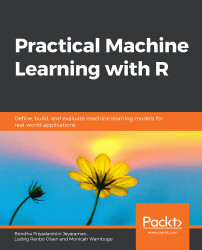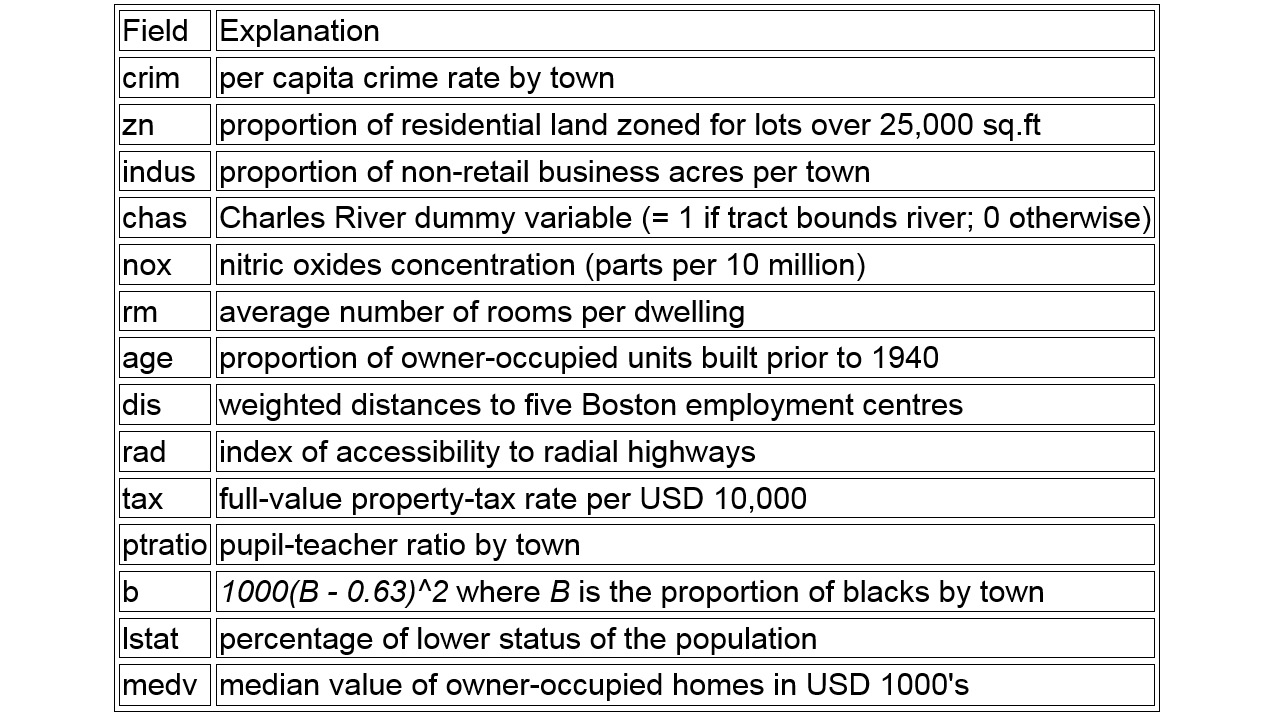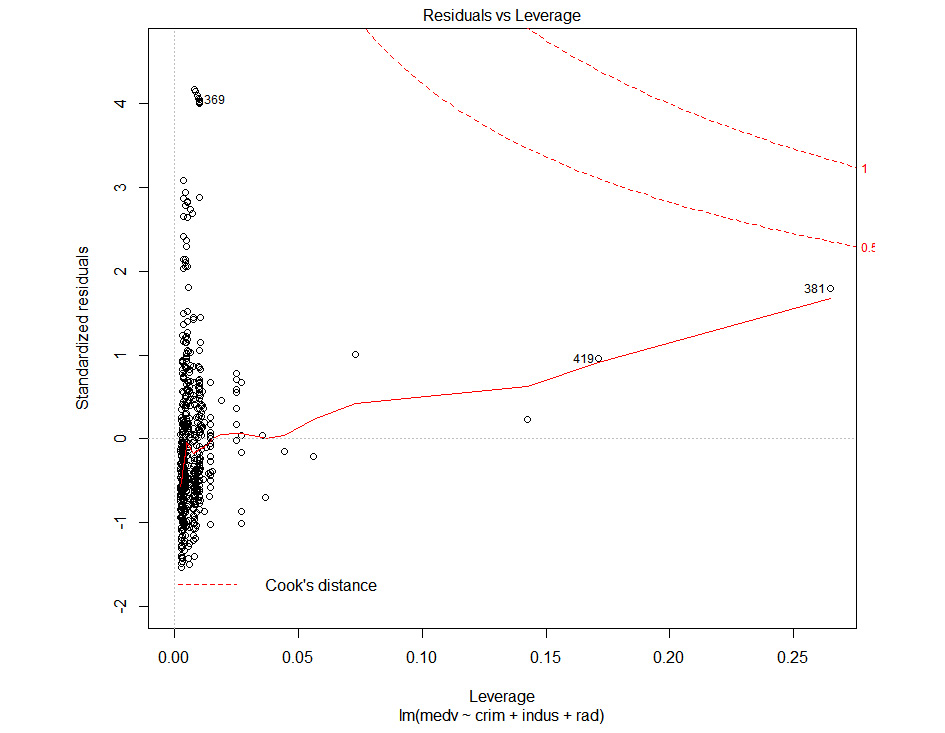•#### Practical Machine Learning with R#### Overview of this book

With huge amounts of data being generated every moment, businesses need applications that apply complex mathematical calculations to data repeatedly and at speed. With machine learning techniques and R, you can easily develop these kinds of applications in an efficient way. Practical Machine Learning with R begins by helping you grasp the basics of machine learning methods, while also highlighting how and why they work. You will understand how to get these algorithms to work in practice, rather than focusing on mathematical derivations. As you progress from one chapter to another, you will gain hands-on experience of building a machine learning solution in R. Next, using R packages such as rpart, random forest, and multiple imputation by chained equations (MICE), you will learn to implement algorithms including neural net classifier, decision trees, and linear and non-linear regression. As you progress through the book, you’ll delve into various machine learning techniques for both supervised and unsupervised learning approaches. In addition to this, you’ll gain insights into partitioning the datasets and mechanisms to evaluate the results from each model and be able to compare them. By the end of this book, you will have gained expertise in solving your business problems, starting by forming a good problem statement, selecting the most appropriate model to solve your problem, and then ensuring that you do not overtrain it.Free Chapter
An Introduction to Machine LearningData Cleaning and Pre-processingFeature EngineeringIntroduction to neuralnet and Evaluation MethodsLinear and Logistic Regression ModelsUnsupervised LearningAppendix## Regression

In this section, we will cover linear regression with single and multiple variables. Let's implement a linear regression model in R. We will predict the median value of an owner-occupied house in the Boston Housing dataset.

The Boston Housing dataset contains the following fields:###### Figure 1.34: Boston Housing dataset fields

Here is a model for the indus field.

#Build a simple linear regression

model1 <- lm(medv~indus, data = BostonHousing)

#summary(model1)

AIC(model1)

The output is as follows:

 3551.601

Build a model considering the age and dis fields:

model2 = lm(medv ~ age + dis, BostonHousing)

summary(model2)

AIC(model2)

Call:

lm(formula = medv ~ age + dis, data = BostonHousing)

Residuals:

Min      1Q  Median      3Q     Max

-15.661  -5.145  -1.900   2.173  31.114

Coefficients:

Estimate Std. Error t value Pr(>|t|)

(Intercept)  33.3982     2.2991  14.526  < 2e-16 ***

age          -0.1409     0.0203  -6.941  1.2e-11 ***

dis          -0.3170     0.2714  -1.168    0.243

---

Signif. codes:  0 ‘***’ 0.001 ‘**’ 0.01 ‘*’ 0.05 ‘.’ 0.1 ‘ ’ 1

Residual standard error: 8.524 on 503 degrees of freedom

Multiple R-squared:  0.1444,    Adjusted R-squared:  0.141

F-statistic: 42.45 on 2 and 503 DF,  p-value: < 2.2e-16

The output is as follows:

 3609.558

AIC is the Akaike information criterion, denoting that the lower the value, the better the model performance. Therefore, the performance of model1 is superior to that of model2.

In a linear regression, it is important to find the distance between the actual output values and the predicted values. To calculate RMSE, we will find the square root of the mean of the squared error using sqrt(sum(error^2)/n).

We have learned to build various regression models with single or multiple fields in the preceding example.

Another type of supervised learning is classification. In the next exercise we will build a simple linear classifier, to see how similar that process is to the fitting of linear regression models. After that, you will dive into building more regression models in the activities.

### Exercise 5: Building a Linear Classifier in R

In this exercise, we will build a linear classifier for the GermanCredit dataset using a linear discriminant analysis model.

The German Credit dataset contains the credit-worthiness of a customer (whether the customer is 'good' or 'bad' based on their credit history), account details, and so on. The dataset can be found at https://github.com/TrainingByPackt/Practical-Machine-Learning-with-R/blob/master/Data/GermanCredit.csv.

library(caret)

data(GermanCredit)

#OR

2. Subset the dataset:

#Subset the data

GermanCredit_Subset=GermanCredit[,1:10]

3. Find the fit model:

# fit model

fit <- lda(Class~., data=GermanCredit_Subset)

4. Summarize the fit:

# summarize the fit

summary(fit)

The output is as follows:

Length Class  Mode

prior    2     -none- numeric

counts   2     -none- numeric

means   18     -none- numeric

scaling  9     -none- numeric

lev      2     -none- character

svd      1     -none- numeric

N        1     -none- numeric

call     3     -none- call

terms    3     terms  call

xlevels  0     -none- list

5. Make predictions.

# make predictions

predictions <- predict(fit, GermanCredit_Subset[,1:10],allow.new.levels=TRUE)\$class

6. Calculate the accuracy of the model:

# summarize accuracy

accuracy <- mean(predictions == GermanCredit_Subset\$Class)

7. Print accuracy:

accuracy

The output is as follows:

 0.71

In this exercise, we have trained a linear classifier to predict the credit rating of customers with an accuracy of 71%. In chapter 4, Introduction to neuralnet and Evaluation Methods, we will try to beat that accuracy, and investigate whether 71% is actually a good accuracy for the given dataset.

### Activity 4: Building Linear Models for the GermanCredit Dataset

In this activity, we will implement a linear regression model on the GermanCredit dataset. The dataset can be found at https://github.com/TrainingByPackt/Practical-Machine-Learning-with-R/blob/master/Data/GermanCredit.csv.

2. Subset the data.
3. Fit a linear model for predicting Duration using lm().
4. Summarize the results.
5. Use predict() to predict the output variable in the subset.
6. Calculate Root Mean Squared Error.

Expected output: In this activity, we expect an RMSE value of 76.3849.

#### Note

The solution for this activity can be found on page 319.

In this activity, we have learned to build a linear model, make predictions on new data, and evaluate performance using RMSE.

### Activity 5: Using Multiple Variables for a Regression Model for the Boston Housing Dataset

In this activity, we will build a regression model and explore multiple variables from the dataset.

Refer to the example of linear regression performed with one variable and use multiple variables in this activity.

The dataset can be found at https://github.com/TrainingByPackt/Practical-Machine-Learning-with-R/blob/master/Data/BostonHousing.csv.

2. Build a regression model using multiple variables.
3. View the summary of the built regression model.
4. Plot the regression model using the plot() function.

The final graph for the regression model will look as follows:#### Note

The solution for this activity can be found on page 320.

We have now explored the datasets with one or more variables.